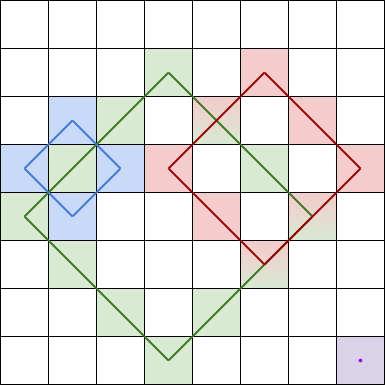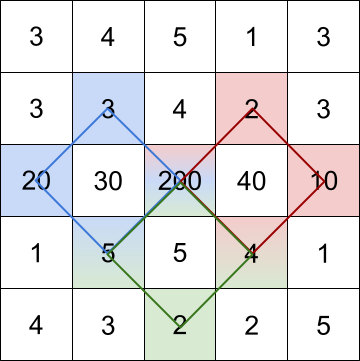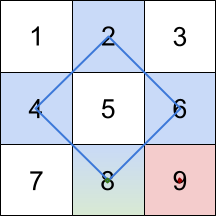Formatted question description: https://leetcode.ca/all/1878.html

# 1878. Get Biggest Three Rhombus Sums in a Grid

Medium

## Description

You are given an m x n integer matrix grid.

A rhombus sum is the sum of the elements that form the border of a regular rhombus shape in grid. The rhombus must have the shape of a square rotated 45 degrees with each of the corners centered in a grid cell. Below is an image of four valid rhombus shapes with the corresponding colored cells that should be included in each rhombus sum:Note that the rhombus can have an area of 0, which is depicted by the purple rhombus in the bottom right corner.

Return the biggest three distinct rhombus sums in the grid in descending order. If there are less than three distinct values, return all of them.

Example 1:Input: grid = [[3,4,5,1,3],[3,3,4,2,3],[20,30,200,40,10],[1,5,5,4,1],[4,3,2,2,5]]

Output: [228,216,211]

Explanation: The rhombus shapes for the three biggest distinct rhombus sums are depicted above.

• Blue: 20 + 3 + 200 + 5 = 228
• Red: 200 + 2 + 10 + 4 = 216
• Green: 5 + 200 + 4 + 2 = 211

Example 2:Input: grid = [[1,2,3],[4,5,6],[7,8,9]]

Output: [20,9,8]

Explanation: The rhombus shapes for the three biggest distinct rhombus sums are depicted above.

• Blue: 4 + 2 + 6 + 8 = 20
• Red: 9 (area 0 rhombus in the bottom right corner)
• Green: 8 (area 0 rhombus in the bottom middle)

Example 3:

Input: grid = [[7,7,7]]

Output: 

Explanation: All three possible rhombus sums are the same, so return .

Constraints:

• m == grid.length
• n == grid[i].length
• 1 <= m, n <= 100
• 1 <= grid[i][j] <= 10^5

## Solution

Loop over all possible rhombuses. For each rhombus, calculate the rhombus sum. Use a tree set to store the rhombus sums and only keep the three biggest rhombus sums. Finally, use an array to store the biggest rhombus sums in descending order and return.

class Solution {
public int[] getBiggestThree(int[][] grid) {
int rows = grid.length, columns = grid.length;
int maxSide = (Math.min(rows, columns) + 1) / 2;
TreeSet<Integer> set = new TreeSet<Integer>();
for (int i = 0; i < rows; i++) {
for (int j = 0; j < columns; j++) {
if (set.size() > 3)
set.remove(set.first());
}
}
for (int side = 2; side <= maxSide; side++) {
int rowStart = 0, rowEnd = rows - (side * 2 - 1);
int columnStart = side - 1, columnEnd = columns - side;
for (int i = rowStart; i <= rowEnd; i++) {
for (int j = columnStart; j <= columnEnd; j++) {
int sum = getSum(grid, i, j, side);
if (set.size() > 3)
set.remove(set.first());
}
}
}
List<Integer> list = new ArrayList<Integer>(set);
Collections.reverse(list);
int size = list.size();
int[] biggest = new int[size];
for (int i = 0; i < size; i++)
biggest[i] = list.get(i);
return biggest;
}

public int getSum(int[][] grid, int topRow, int topColumn, int side) {
int bottomRow = topRow + (side - 1) * 2;
int sum = grid[topRow][topColumn] + grid[bottomRow][topColumn];
for (int i = 1; i < side; i++)
sum += grid[topRow + i][topColumn - i] + grid[topRow + i][topColumn + i];
for (int i = 1; i < side - 1; i++)
sum += grid[bottomRow - i][topColumn - i] + grid[bottomRow - i][topColumn + i];
return sum;
}
}